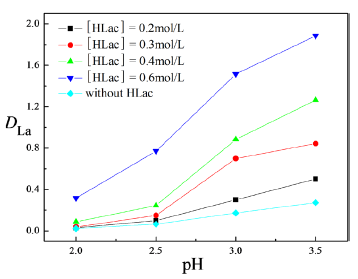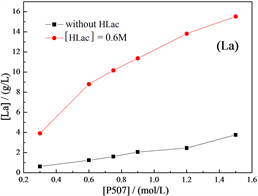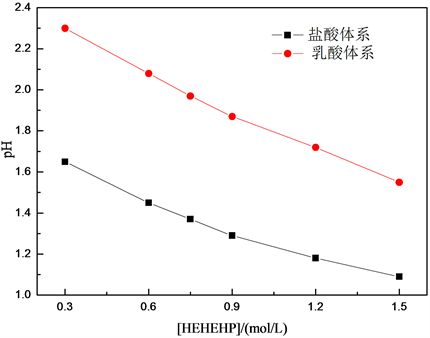# 乳酸在水溶液中对HEHEHP-La-HCl体系的络合助萃行为Complexation of Lactic Acid to HEHEHP-La-HCl System in Aqueous Solution

• 全文下载: PDF(749KB)    PP.18-22   DOI: 10.12677/AAC.2019.91003
• 下载量: 213  浏览量: 498   科研立项经费支持

Rare earth is a national strategic resource, its separation and purification is the focus of attention at home and abroad. This paper presents a new type of chelating extraction separation technology, by adding complexing agent (HLac) into aqueous phase to improve the existing extraction of rare earth La. The experimental results shown that HLac can be combined with La, and in the process of aqueous phase and organic phase mixing together, it rereacted with the extractant to generate rare earth chelate. In the process of extraction, the complexation of HLac can buffer the influence of H+ in aqueous phase, and eventually improve the extraction performance of La in non-saponification HEHEHP (2-ethyl hexyl phosphonic acid single 2-ethyl hexyl ester) extracting system.

1. 引言

2. 实验过程

$\text{D=}\frac{{\text{C}}_{有}}{{\text{C}}_{水}}$ (1)

3. 实验结果与讨论

3.1. 乳酸在水相中的存在形式

${\text{RE}}_{\text{2}}{\text{O}}_{\text{3}}+6{\text{H}}^{+}+3{\text{Lac}}^{-}=\text{RE}{\left(\text{Lac}\right)}_{{}_{\text{2}}}^{+}+\text{RE}{\left(\text{Lac}\right)}^{2+}+{\text{3H}}_{\text{2}}\text{O}$ (2)

3.2. 乳酸对萃取过程的助萃效果Figure 1. The relationship between DLa and the pH value of the feed solution when extracting La with HEHEHP at different lactic acid concentrationsFigure 2. The relationship between HEHEHP concentration and the amount of La(III) extraction from chlorid medium

3.3. 乳酸在萃取过程中对萃余液酸度的缓冲作用Figure 3. The equilibrium pH value when extracting La with HEHEHP in hydrochloric acid system and lactic acid system

3.4. 乳酸在萃取过程中的循环水解作用

${\text{La}}^{\text{3}+}{}_{\text{(a)}}+{\text{H}}_{\text{2}}{\text{L}}_{\text{2}}{}_{\text{(o)}}\stackrel{\text{K}}{↔}\text{RE}{\left({\text{HL}}_{\text{2}}\right)}_{\text{3}}{}_{\text{(o)}}+{\text{3H}}^{+}$ (3)

HLac = H+ + Lac-(4)

${\text{La}}_{\text{2}}{\text{O}}_{\text{3}}+6{\text{H}}^{+}+3{\text{Lac}}^{-}=\text{La}{\left(\text{Lac}\right)}_{{}_{\text{2}}}^{+}+\text{La}{\left(\text{Lac}\right)}^{2+}+{\text{3H}}_{\text{2}}\text{O}$ (5)

$\text{La}{\left(\text{Lac}\right)}^{\text{2}+}{}_{\text{(a)}}+\text{La}{\left(\text{Lac}\right)}_{\text{2}}^{+}{}_{\text{(a)}}+6{\text{H}}_{\text{2}}{\text{L}}_{\text{2}}{}_{\text{(o)}}\to 2\text{La}{\left({\text{HL}}_{\text{2}}\right)}_{\text{2}}{}_{\text{(o)}}+{\text{3H}}^{+}{}_{\text{(a)}}+{\text{3HLac}}_{\text{(a)}}$ (6)

${\text{La}}_{\text{2}}{\text{O}}_{\text{3}}{}_{\left(\text{a}\right)}+6{\text{H}}^{+}{}_{\left(\text{a}\right)}={\text{La}}^{3+}{}_{\left(\text{a}\right)}+{\text{3H}}_{\text{2}}{\text{O}}_{\left(\text{a}\right)}$ (7)

$2{\text{La}}^{\text{3}+}{}_{\left(\text{a}\right)}+{\text{3Lac}}^{-}{}_{\left(\text{a}\right)}=\text{La}{\left(\text{Lac}\right)}^{\text{2}+}{}_{\left(\text{a}\right)}+\text{La}{\left(\text{Lac}\right)}_{\text{2}}^{+}$ (8)

$\text{La}{\left(\text{Lac}\right)}^{\text{2}+}{}_{\left(\text{a}\right)}+\text{La}{\left(\text{Lac}\right)}_{\text{2}}^{+}{}_{\left(\text{a}\right)}+6{\text{H}}_{\text{2}}{\text{L}}_{\text{2}}{}_{\left(\text{o}\right)}\to 2\text{La}{\left({\text{HL}}_{\text{2}}\right)}_{\text{2}}{}_{\left(\text{o}\right)}+{\text{3H}}^{+}{}_{\left(\text{a}\right)}+{\text{3HLac}}_{\left(\text{a}\right)}$ (9)

${\text{La}}_{\text{2}}{\text{O}}_{\text{3}}+6{\text{H}}^{+}+3{\text{Lac}}^{-}=\text{La}{\left(\text{Lac}\right)}_{{}_{\text{2}}}^{+}+\text{La}{\left(\text{Lac}\right)}^{2+}+{\text{3H}}_{\text{2}}\text{O}$ (10)

……

4. 结论

1) 乳酸通过络合水解作用可以中和阳离子交换产生的H+，缓冲酸度对萃取过程的影响，是一种绿色萃取新技术，从源头上杜绝了皂化废水的产生，达到了高效萃取La的目的。

2) 与盐酸体系相比，乳酸的助萃效应可以提高稀土La的萃取量和分配比，当乳酸浓度为0.6mol/L时萃取效果最好。

  张璞, 李毅. 稀土产业现状与资源整合重组对策[J]. 稀土, 2013, 34(1): 94-97.  王登红, 赵芝, 于扬, 等. 离子吸附型稀土资源研究进展、存在问题及今后研究方向[J]. 岩矿测试, 2013, 32(5): 796-802.  黄小卫. HDEHP-HEH/EHP-H2SO4体系协同萃取分离稀土的研究[D]: [博士学位论文]. 沈阳: 东北大学, 2008.  Juang, R.S. and Wang, Y.C. (2003) Use of Complexing Agents for Effective Ion-Exchange Separation of Co(II)/Ni(II) from Aqueous Solutions. Water Research, 37, 845-852.https://doi.org/10.1016/S0043-1354(02)00423-2  戴猷元, 秦炜, 张瑾, 等. 有机物络合萃取化学[M]. 北京: 化学工业出版社, 2008: 5.  Sun, X.B., Wang, Y.G. and Li, D.Q. (2006) Selective Separation of Yttrium by CA-100 in the Presence of a Complexing Agent. Journal of Alloys and Compounds, 408-412, 999-1002. https://doi.org/10.1016/j.jallcom.2004.12.137  Zhang, F.Y., Dai, J.J., Wang, A.M. and Wu, W.Y. (2017) Investigation of the Synergistic Extraction Behavior between Cerium (III) and Two Acidic Organophosphorus Extractants Using FT-IR, NMR and Mass Spectrometry. Inorganica Chimica Acta, 466, 333-342. https://doi.org/10.1016/j.ica.2017.06.016  Zhang, F.Y., Wang, A.M., Zhou, F.M., Dai, J.J. and Wu, W.Y. (2017) Behavior of a Synergistic System in the Extrac-tion of Pr(III) from Chloride Medium Using di-2-Ethylhexyl Phosphoric Acid and 2-Ethylhexylphosphonic Mono-2-Ethylhexyl Ester. Separation Science and Technology, 52, 1015-1021. https://doi.org/10.1080/01496395.2017.1281301  Jiang, F., Yin, S.H., Srinivasakannan, C., Li, S.W. and Peng, J.H. (2018) Separation of Lanthanum and Cerium from Chloride Medium in Presence of Complexing Agent along with EHEHPA (P507) in a Serpentine Microreactor. Chemical Engineering Journal, 334, 2208-2214. https://doi.org/10.1016/j.cej.2017.11.173  徐光宪, 袁成业. 稀土的溶剂萃取[M]. 北京: 科学出版社, 2010.  Ahmed, A.A., Susumu, N., Fumio, K. and Katsuroku, T. (2002) Separation of La and Ce with PC-88A by Coun-ter-Current Mixer-Settler Extraction Column. Separation and Purification Technology, 26, 265-272. https://doi.org/10.1016/S1383-5866(01)00180-0